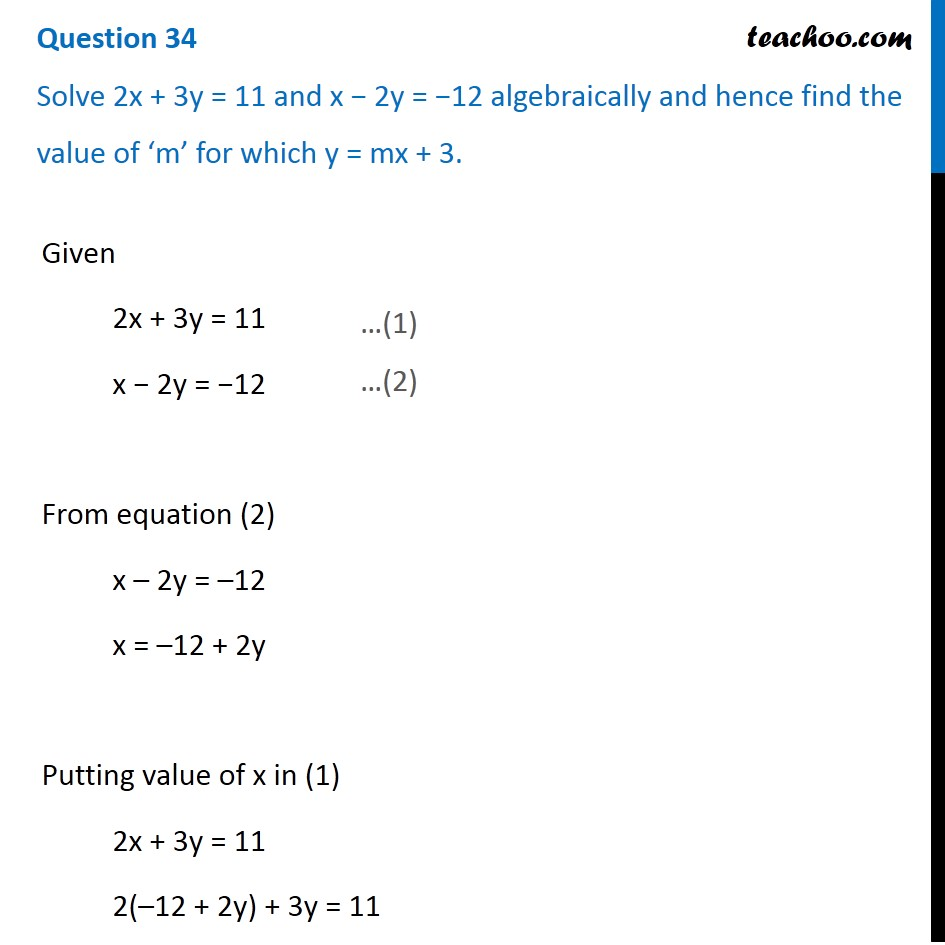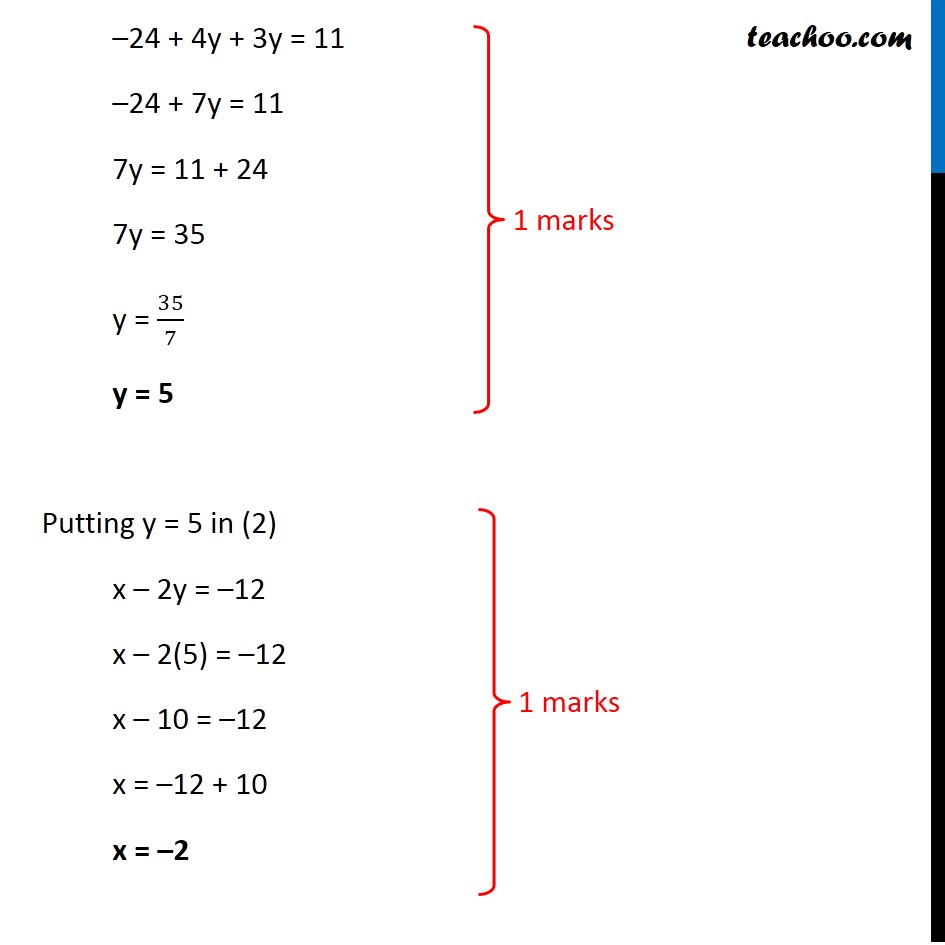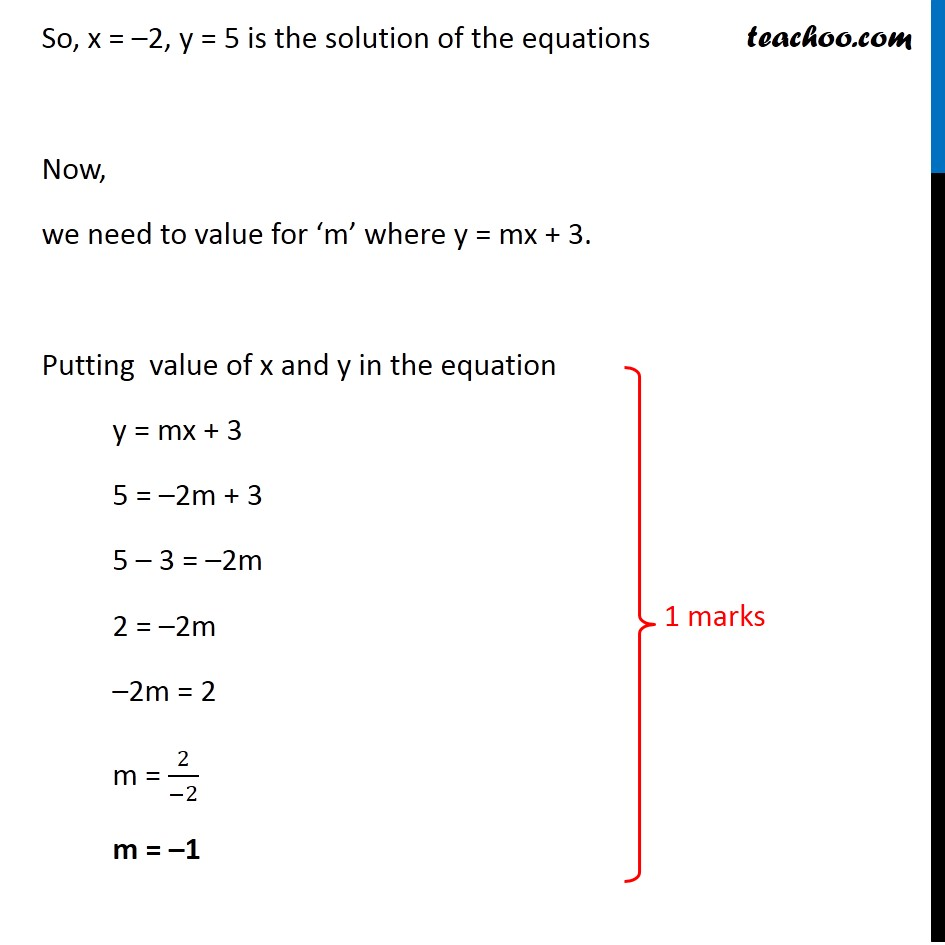## Solve 2x + 3y = 11 and x − 2y = −12 algebraically and hence find the value of ‘m’ for which y = mx + 3.1. Class 10
2. Solutions of Sample Papers for Class 10 Boards
3. CBSE Class 10 Sample Paper for 2020 Boards - Maths Basic

Transcript

Question 34 Solve 2x + 3y = 11 and x − 2y = −12 algebraically and hence find the value of ‘m’ for which y = mx + 3. Given 2x + 3y = 11 x − 2y = −12 From equation (2) x – 2y = –12 x = –12 + 2y Putting value of x in (1) 2x + 3y = 11 2(–12 + 2y) + 3y = 11 –24 + 4y + 3y = 11 –24 + 7y = 11 7y = 11 + 24 7y = 35 y = 35/7 y = 5 Putting y = 5 in (2) x – 2y = –12 x – 2(5) = –12 x – 10 = –12 x = –12 + 10 x = –2 So, x = –2, y = 5 is the solution of the equations Now, we need to value for ‘m’ where y = mx + 3. Putting value of x and y in the equation y = mx + 3 5 = –2m + 3 5 – 3 = –2m 2 = –2m –2m = 2 m = 2/(−2) m = –1

CBSE Class 10 Sample Paper for 2020 Boards - Maths Basic

Class 10
Solutions of Sample Papers for Class 10 Boards